# Chapter 04:Shear and Moment in Beams¶

## Example 4.4.1, Page No:103¶

In :
import math
%matplotlib  inline

#Variable Decleration
F1=14 #Force in kN
F2=28 #Force in kN
l1=2 #Length in m
l2=3 #Length in m
Ra=18 #Reaction at point A in kN
Rb=24 #Reaction at point D in kN

#Calculations
#Part(1)
#Applying the summation of force in y in segment AB
V_ab=Ra # Shear in part AB in kN
#Moment is in the variable form M=18x kN.m
#Segment BC
V_bc=Ra-F1 #Shear in the segment BC in kN
#Moment in the form M=4x+28 kN.m
#Segment CD
V_cd=Ra-F1-F2 #Shear in the segment CD in kN
#Moment in the form -24x+168 kN.m

#Importing the plotting libraries and computing the plots
import matplotlib.pyplot as plt

#Result
print "The Shear Force and Bending Moment Diagrams are the results"
#Plotting the SHear Force Diagram

X1=[0,l1,l1+0.0000000001,l1+l2,l1+l2+0.0000000001,l1+l2+l1]
Y1=[V_ab,V_ab,V_bc,V_bc,V_cd,V_cd]
Z1=[0,0,0,0,0,0]
plt.plot(X1,Y1,X1,Z1)
plt.xlabel("Length x in m")
plt.ylabel("Shear Force in kN")
plt.show()

#Plotting the Bendimg Moment Diagram

Y2=[0,36,48,0]
X2=[0,l1,l1+l2,l1+l2+l1]
Z2=[0,0,0,0]
plt.plot(X2,Y2)
plt.xlabel("Lenght in m")
plt.ylabel("Bending Moment in kN.m")
plt.show()

The Shear Force and Bending Moment Diagrams are the results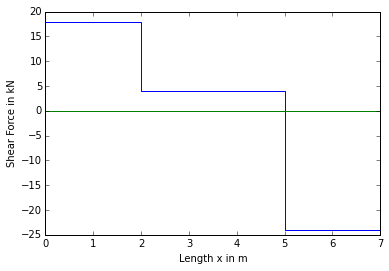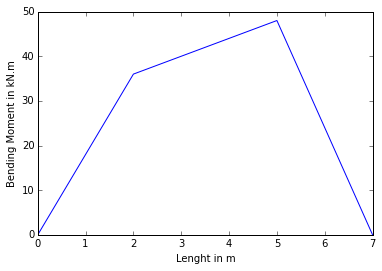## Example 4.4.3, Page No:108¶

In :
import math
import numpy as np
import matplotlib.pyplot as plt
%matplotlib inline

#Variable Decleration
L=12 #Length of the beam in ft
F=1000 #Force at the tip of the beam in lb
#w=30x Force per length on the beam in lb/ft
a=15 #Constant

#Calculations
#Part(1)
#Here all the computation is in variable form
#Applying the sum of forces
#V=1000-15x^2
#Applying moment about C
#M=1000x-5x^3

#Part(2)
#Max BM when shear force is zero
x=(F*a**-1)**0.5 #Length at which BM is max in ft
M_max=F*x-5*x**3 #Max BM in lb.ft

#Result
b=np.linspace(0,L,20) #Array
c=np.linspace(0,0,20) #Zero array
V=F-(15*b**2) #Shear Force
M=F*b-5*b**3 #Bending Moment

#Shear force plot
plt.plot(b,V,b,c)
plt.xlabel("Length in ft")
plt.ylabel("Shear Force in lb")
plt.show()

#Bending Moment plot
plt.plot(b,M)
plt.xlabel("Length in ft")
plt.ylabel("Bending Moment in lb.ft")
plt.show()

print "The maximum BM is",round(M_max),"lb.ft"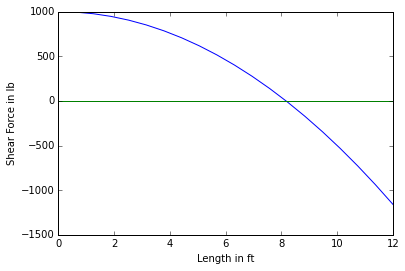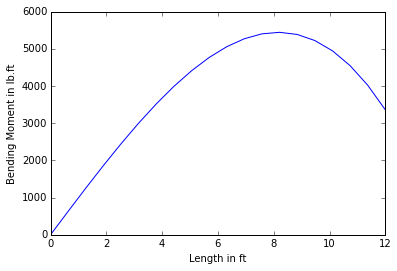The maximum BM is 5443.0 lb.ft


## Example 4.4.4, Page no:117¶

In :
import math
import numpy as np
import matplotlib.pyplot as plt

#Variable Decleration
P=30 #Force in kN
M=40 #Moment in kN.m
L1=3 #Length in m
L2=4 #Length in m
Ra=14 #Reaction at A in kN
Re=16 #Reaction at E in kN

#Calculations
#The plotting will done different from that done in the textbook
#Plot for Shear Force
x_shear=[0,0.000000001,L2,L2+0.000000001,L2+L1,L2+L1*2,L2+L1*2+0.00000001]
y_shear=[0,Ra,Ra,Ra-P,Ra-P,Ra-P,0]
#Plot for Bending Moment
x_bm=[0,L2,L2+L1,L2+L1+0.00000001,L2+L1+L1]
y_bm=[0,Ra*L2,Ra*(L2+L1)-P*L1,Ra*(L2+L1)-P*L1+M,0]
#Zero line
x1=[0,0,0,0,0,0,0]

#Result
print "The plots below are the answers"

plt.plot(x_shear,y_shear,x_shear,x1)
plt.xlabel("Distance from point A in m")
plt.ylabel("Shear Force in kN")
plt.show()

plt.plot(x_bm,y_bm)
plt.xlabel("Distance from point A in m")
plt.ylabel("Bending Moment in kN.m")
plt.show()

The plots below are the answers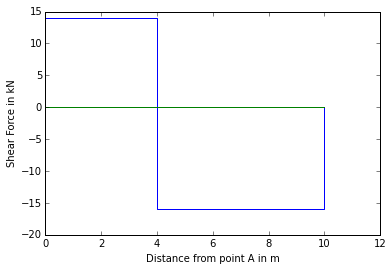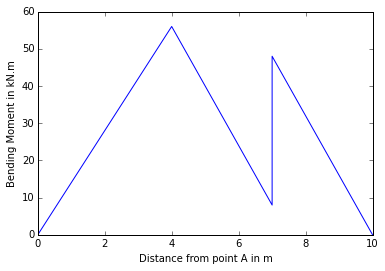## Example 4.4.5, Page No:119¶

In :
import math
import matplotlib.pyplot as plt
%matplotlib inline

#Variable Decleration
w1=400 #UDL in lb/ft
P=400 #Point load at C in lb
w2=200 #UDL in lb/ft
L1=2 #Length in ft
L2=1 #Length in ft
L3=4 #Length in ft
V_A=0 #Shear force at A in lb
Rb=-1520 #Reaction at B in lb
Rd=-880 #Reaction at D in lb
d=1.6 #Distance in ft

#Calculations
#The plotting of the Shear force diagram and the Bending Moment Diagram
#Will be done different from that done in the textbook

#Calculations for Shear Force
Area1=P*L1 #Area of w diagram from A to B
Area2=0 #Area of w diagram from B to C
Area3=w2*L3 #Area of w diagram from C to D
V_B_left=V_A-Area1 #Shear Force at left of B in lb
V_B_right=V_B_left-Rb #Shear force at right of B in lb
V_C_left=V_B_right-Area2 #Shear Force at left of C in lb
V_C_right=V_C_left-P #Shear Force at right of C in lb
V_D_left=V_C_right-Area3 #Shear Force at left of D in lb
V_D_right=V_D_left-Rd #Shear Force at right of D in lb
V_E=0 #Shear Force at E in lb

#Calculations for Bending Moments
AreaV1=0.5*L1*V_B_left #Area of V diagram
AreaV2=V_C_left*L2 #Area of V diagram from B to C
AreaV3=V_D_left*(L3-d)*0.5 #Area of V diagram from F to D
M_A=0 #Moment at A in lb.ft
M_B=M_A+AreaV1 #Moment about B in lb.ft
M_C=M_B+AreaV2 #Moment about C in lb.ft
M_F=M_C+V_C_right*0.5*d #Moment about F in lb.ft
M_D=M_F+AreaV3 #Moment about D in lb.ft
M_E=0 #Moment about E in lb.ft

#Result
print "The following plots are the results"

#Plotting

#Shear Force
x=[0,L1,L1+0.000001,L1+d,L1+d+0.000001,L1+L3,L1+L3+0.000001,L1+L3+L1]
V=[V_A,V_B_left,V_B_right,V_C_left,V_C_right,V_D_left,V_D_right,V_E]
zero=[0,0,0,0,0,0,0,0]
plt.plot(x,V,x,zero)
plt.xlabel("Length in ft")
plt.ylabel("Shear Force in lb")
plt.title("Shear Force Diagram")
plt.show()

#Bending Moment
x1=[0,L1,L1+L2,L1+L2+d,L1+L3,L1+L3+L1]
BM=[M_A,M_B,M_C,M_F,M_D,M_E]
zero1=[0,0,0,0,0,0]
plt.plot(x1,BM,x1,zero1)
plt.xlabel("Length in ft")
plt.ylabel("Bending Moment in lb.ft")
plt.title("Bending Moment Diagram")
plt.show()

#The Bending Moment Diagram differs from that in the textbook because of curve type

The following plots are the results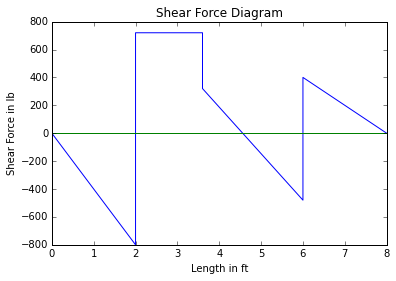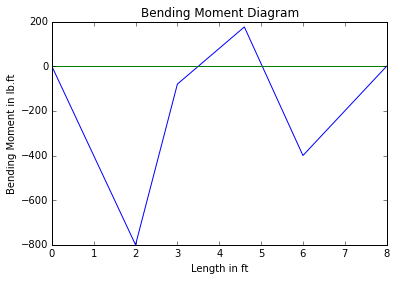## Example 4.4.6, Page No:127¶

In :
import math

#Variable Decleration
P1=15 #Load in kN
P2=25 #Load in kN
P3=50 #Load in kN
R=90 #Load in kN
L1=3.5 #Length in m
L2=2 #Length in m
L3=3 #Length in m
L=12 #Total span in m

#Calculation
#Part 1
#Maximum Bending Moment at A
R1=R*L1*L**-1 #Reaction 1 in kN
M_A=R1*L1 #Moment about A in kN.m
#Maximum Bending Moment at B
R1_2=R*(L1+(L3-L2))*L**-1 #reaction 1 in kN
M_B=R1_2*(L1+(L3-L2))-P1*L2 #Moment at B in kN.m

#Maximum Moment at C
R2=(P2+P3)*(L2+L3)*L**-1 #Reaction 2 in kN
M_C=R2*(L2+L3) #Moment at C in kN.m

M_max=max(M_A,M_B,M_C) #Maximum Bending Moment in kN.m

#Part 2
R2_2=R*(L-L3)*L**-1 #Reaction 2 in kN

V_max=max(R1,R2,R1_2,R2_2) #Maximum Shear Force in kN

#Result
print "The maximum Shear force is",V_max,"kN and the Maximum Bending Moment is",round(M_max,1),"kN.m"

The maximum Shear force is 67.5 kN and the Maximum Bending Moment is 156.3 kN.m